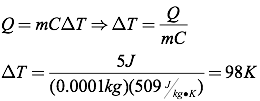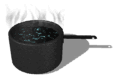# Heat

Heat is the transfer of thermal energy from one object to another object due to a difference in temperature. Heat always flows from warmer objects to cooler objects. The symbol for heat in physics is Q, with positive values of Q representing heat flowing into an object, and negative values of Q representing heat flowing out of an object.

When heat flows into or out of an object, the amount of temperature change depends on the material. The amount of heat required to change one kilogram of a material by one degree Celsius (or one Kelvin) is known as the material’s specific heat (or specific heat capacity), represented by the symbol C.The relationship between heat and temperature is quantified by the following equation, where Q is the heat transferred, m is the mass of the object, C is the specific heat, and ΔT is the change in temperature (in degrees Celsius or Kelvins).Question: A half-carot diamond (0.0001 kg) absorbs five Joules of heat. How much does the temperature of the diamond increase?

Answer:Question: A three-kilogram aluminum pot is filled with five kilograms of water. How much heat is absorbed by the pot and water when both are heated from 25°C to 95°C?

Answer: Using the table of specific heats, you can find the heat added to each item separately, and then combine them to get the total heat added.The total heat absorbed, therefore, must be 1.65×106 Joules.

Question: Two solid metal blocks are placed in an insulated container. If there is a net flow of heat between the blocks, they must have different

1. initial temperatures
2. melting points
3. specific heat
4. heats of fusion

Answer: (1) since heat flows from warmer objects to cooler objects.

Heat can be transferred from one object to another by three different methods: conduction, convection, and radiation. Conduction is the transfer of heat along an object due to the particles comprising the object colliding. When you stick an iron rod in a fire, the end in the fire warms up, but over time, the particles comprising the iron rod near the fire move more quickly, colliding with other particles in the iron speeding them up, and so on, and so on, resulting in heat transfer down the length of the iron rod until the end you’re holding far away from the fire becomes very hot!

Convection, on the other hand, is a result of the energetic (heated) particles moving from one place to another. A great example of this is a convection oven. In a convection oven, air molecules are heated near the burner or electrical element, and then circulated throughout the oven, transferring the heat throughout the entire oven’s volume.

Radiation is the transfer of heat through electromagnetic waves. Think of a campfire or fireplace on a cold evening. When you want to warm up, you place your hands up in front of you, allowing your hands to absorbthe maximum amount of electromagnetic waves (mostly infrared) coming from the fire, making you nice and toasty!Looking more closely at conduction specifically, the rate of heat transfer (H), as measured in Joules per second, or Watts, depends on the magnitude of the temperature difference across the object (ΔT), the cross-sectional area of the object (A), the length of the object (L) and the thermal conductivity of the material (k). Thermal conductivities are typically provided to you in a problem, or you can look them up in a table of thermal conductivities.Question: Find the rate of heat transfer through a 5 mm thick glass window with a cross-sectional area of 0.4 m2 if the inside temperature is 300K and the outside temperature is 250K.

Answer:Question: One end of a 1.5-meter-long stainless steel rod is placed in an 850K fire. The cross-sectional radius of the rod is 1 cm, and the cool end of the rod is at 300K. Calculate the rate of heat transfer through the rod.

Answer: To solve this problem, you must first find the cross-sectional area of the rod.Next, calculate the heat transfer through the rod.